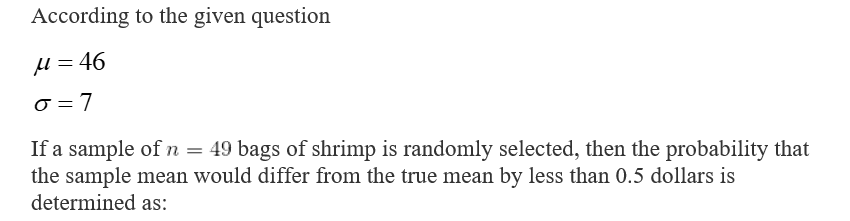# The mean cost of a five pound bag of shrimp is 46 dollars with a standard deviation of 7 dollars.If a sample of 49 bags of shrimp is randomly selected, what is the probability that the sample mean would differ from the true mean by more than  0.5 dollars? Round your answer to four decimal places.

Question
55 views

The mean cost of a five pound bag of shrimp is 46 dollars with a standard deviation of 7 dollars.

If a sample of 49 bags of shrimp is randomly selected, what is the probability that the sample mean would differ from the true mean by more than  0.5 dollars? Round your answer to four decimal places.

check_circle

Step 1...

### Want to see the full answer?

See Solution

#### Want to see this answer and more?

Solutions are written by subject experts who are available 24/7. Questions are typically answered within 1 hour.*

See Solution
*Response times may vary by subject and question.
Tagged in